Home Practice
For learners and parents For teachers and schools
Textbooks
Full catalogue
Pricing SupportLog in

We think you are located in United States. Is this correct?

# 1.4 Squares, square roots and cubes

## 1.4 Squares, square roots and cubes (EMGM)

### Squares (EMGN)

The square of a number is the number multiplied by itself. We can represent squares of numbers in diagrams. The number of blocks along the side of the square is the number that is being squared. The total number of small squares in each diagram is equal to the square of the number.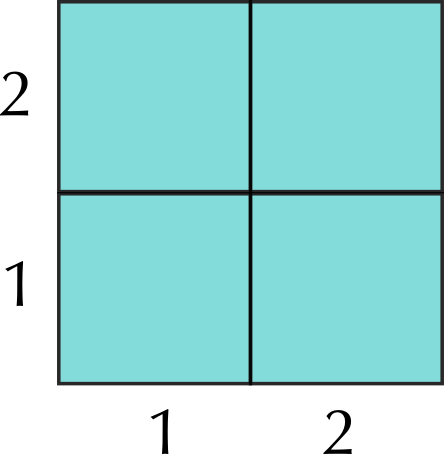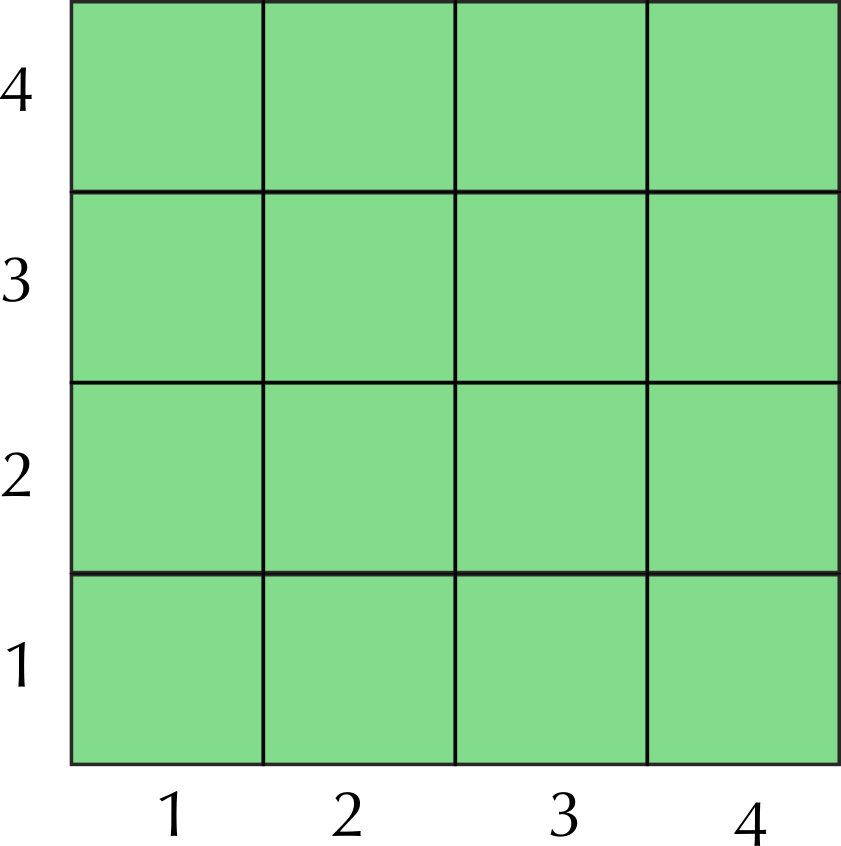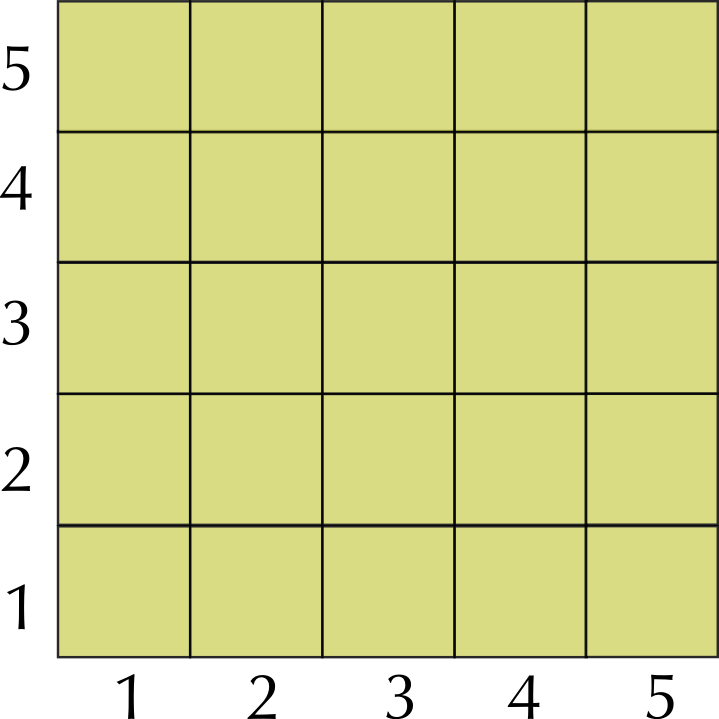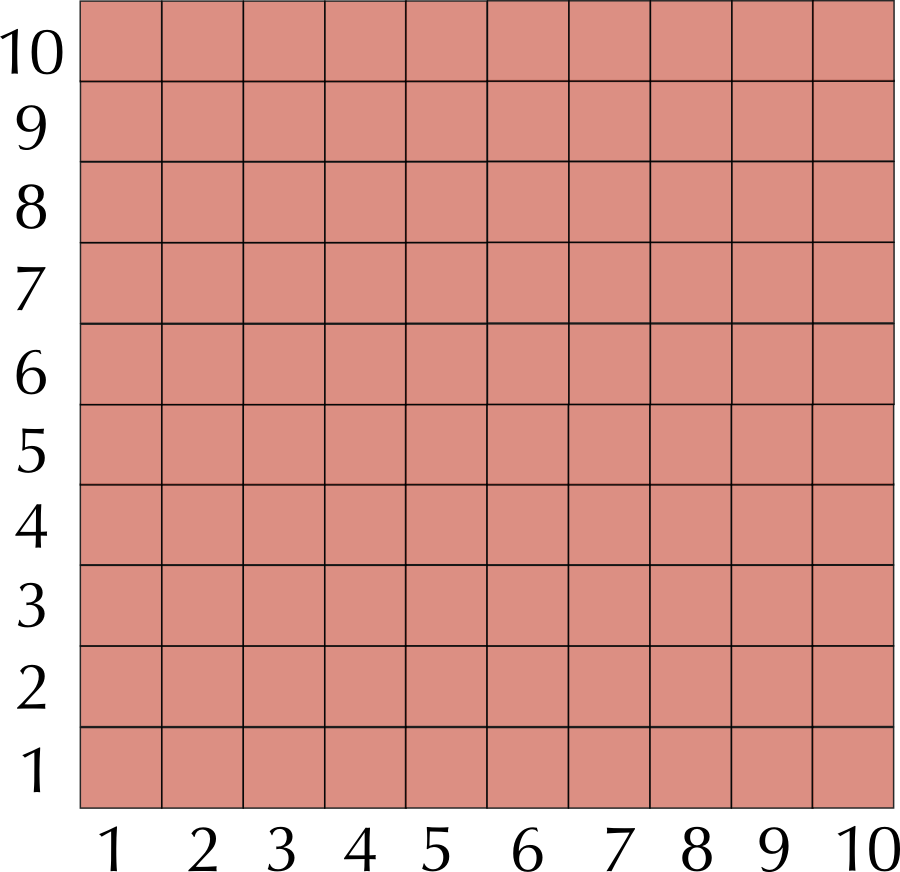Notice that the area of a square is equal to the side squared. $$\text{2} \times \text{2}$$ squares = $$\text{2}^{\text{2}} = \text{4}$$ squares in total, $$\text{4} \times \text{4}$$ squares = $$\text{4}^{\text{2}} = \text{16}$$ squares in total and so on. You need to know how to square numbers in order to work with area in later chapters.

### Square roots (EMGP)

In each case, the number that is squared is the square root. So the square root of the diagram representing $$\text{4}^\text{2}$$ and is equal to $$\text{4}$$. We can write this as $$\sqrt{\text{16}}= \text{4}$$.

Notice that finding the square root of a number is the same as finding the side of the square. It is the opposite to squaring the number.

### Cubes (EMGQ)

In the same way, a number to the power of three is called the cube of the number. So $$\text{3}^\text{3}$$ is $$\text{3} \times \text{3} \times \text{3}$$, or three cubed and is equal to $$\text{27}$$. The length of each side is equal to the number that is cubed.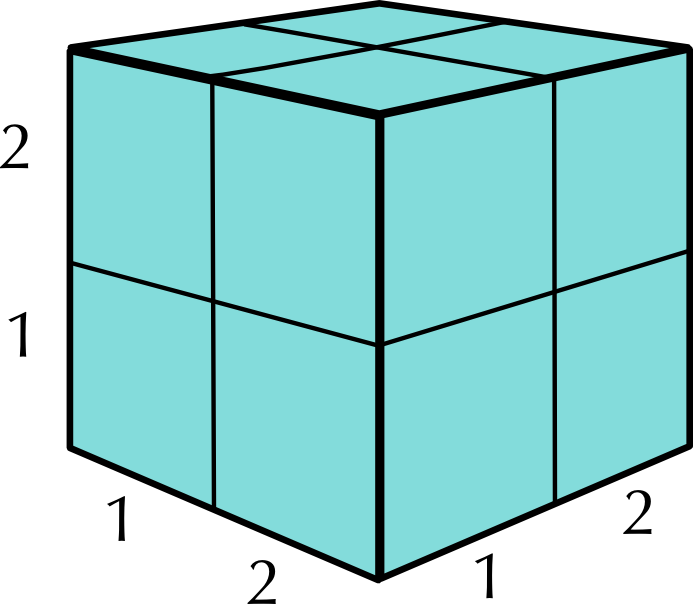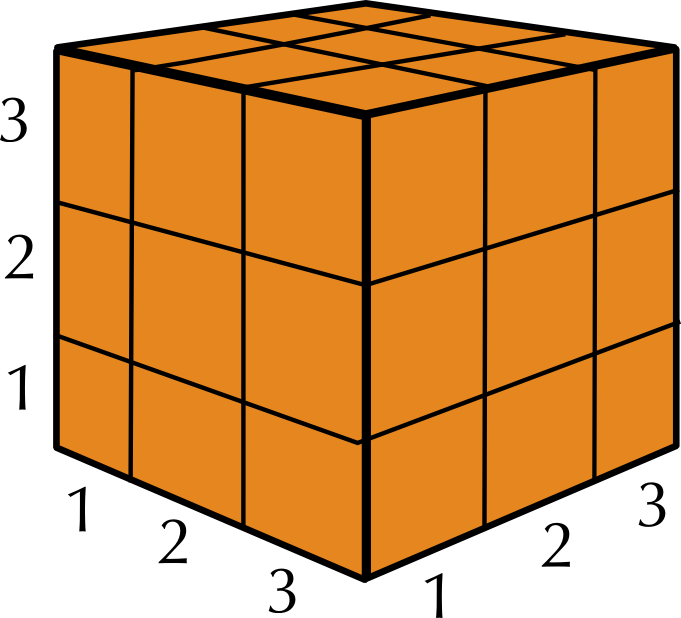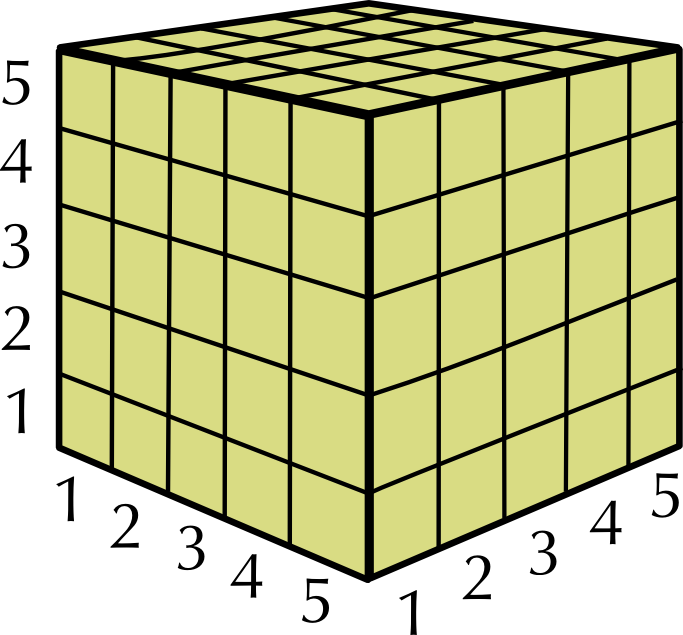To square a number, multiply it by itself, e.g. $$\text{2} \times \text{2} = \text{2}^{\text{2}}= \text{4}$$.

To cube a number, multiply it by itself twice, e.g. $$\text{2} \times \text{2} \times \text{2} = \text{2}^{\text{3}} = \text{8}$$.

It is also easy to work out square roots on your calculator; simply enter the number and then the square root key.

## Squares, square roots and cubes

Exercise 1.6

Use you calculator to work out the following squares:

$$\text{200}^\text{2}$$

$$\text{40 000}$$

$$\text{413}^\text{2}$$

$$\text{170 569}$$

$$\text{3 100}^\text{2}$$

$$\text{9 610 000}$$

$$\text{2 567}^\text{2}$$

$$\text{6 589 489}$$

A tile shop has tiles of various sizes for sale. Calculate the length of the sides of square tiles with the following areas:

$$\text{121}$$ $$\text{cm}$$$$^\text{2}$$

$$\text{11}$$ $$\text{cm}$$

$$\text{625}$$ $$\text{cm}$$$$^\text{2}$$

$$\text{25}$$ $$\text{cm}$$

$$\text{400}$$ $$\text{cm}$$$$^\text{2}$$

$$\text{20}$$ $$\text{cm}$$

$$\text{14 400}$$ $$\text{mm}$$$$^\text{2}$$

$$\text{120}$$ $$\text{mm}$$

Calculate the volumes of cubes which have sides of these lengths:

$$\text{14}$$ $$\text{mm}$$

$$\text{14}^\text{3} = \text{2 744}$$ mm$$^{\text{3}}$$

$$\text{28}$$ $$\text{mm}$$

$$\text{28}^\text{3} = \text{21 952}$$ mm$$^{\text{3}}$$

$$\text{105}$$ $$\text{mm}$$

$$\text{105}^\text{3} = \text{1 157 625}$$ mm$$^{\text{3}}$$

$$\text{81}$$ $$\text{cm}$$

$$\text{81}^\text{3} = \text{531 441}$$ cm$$^{\text{3}}$$